# What Is an Equation?

An equation shows a relationship between two quantities. Here is a simple example:The equals sign separates an equation into two parts. For this equation, the left side has “1+1” and the right side has “2”.

# SeeSaw Model for an Equation

Here is a visual way to show an equation using a seesaw as a visual model:The equal sign is the middle part of the seesaw, the fulcrum. Each side of the equation is on one side of the seesaw. When an equation is true, the seesaw is balanced.

Here’s another representation of the equation.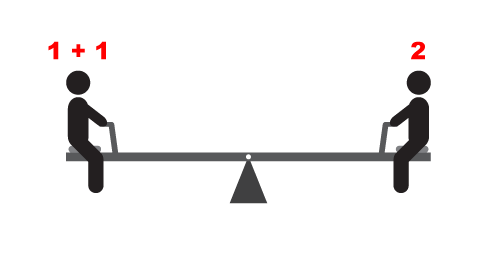# “Scale” Model for an Equation

Now look at this model for an equation.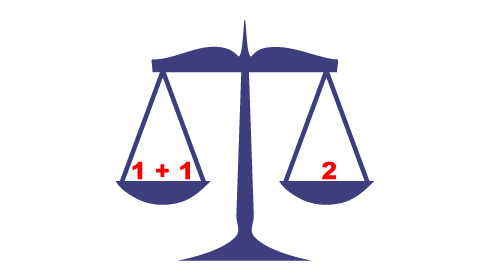This is a balance scale. The expressions on either side of the equals sign are like “weights”. A balanced scale means that the equation is a true equation.

Here’s an example of a false equation.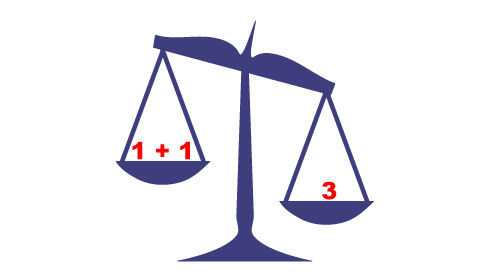You can see that 3 “weighs” more than “1+1”. Therefore, an unbalanced equation is a false equation.

# Modeling Equations with Unknowns

Look at this visual model.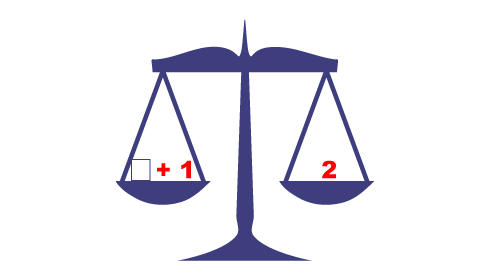This is an example of a conditional equation. In other words, for what value(s) is this a true equation?

As you can see, it is only when the value in the box (the “unknown”) is 1 does the equation become true.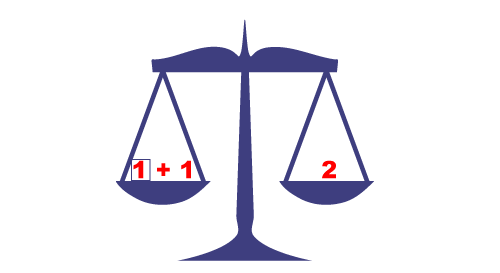# Modeling Equations with Algebra Tiles

Algebra Tiles are useful for modeling and solving equations. With Algebra tiles, you can model the variable, x, positive integers, and negative integers.Let’s look at an example of an equation using Algebra Tiles.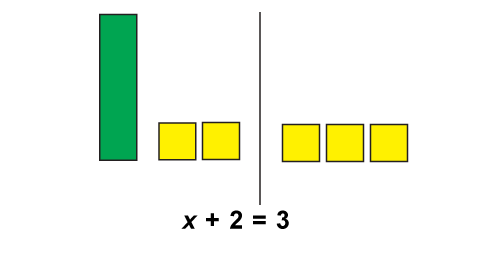The tiles are used to model the equation x + 2 = 3. The green x-tile and the two yellow tiles model the left side of the equation (x + 2). The three yellow tiles model the right side of the equation (3). The vertical bar separates the two sets of tiles and models the equals sign.

To solve this equation, isolate the variable. This means removing the two positive integers from the left side. Here’s how to do it.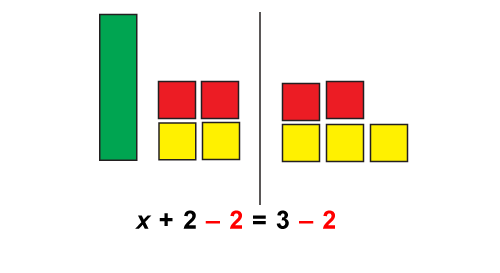Adding two negative integers to each side of the equation is the same as subtracting 2 from both sides. This is an example of the Subtraction Property of Equality. By doing the same operation to both sides of the equation (addition, subtraction, multiplication, or division) doesn’t change the equality.

The solution to the equation is shown here.